Home / Blog / Data Science / Introduction to SciPy, Installation, SciPy in Python

# Introduction to SciPy, Installation, SciPy in Python

• July 28, 2023
• 2416
• 71### Meet the Author : Mr. Bharani Kumar

Bharani Kumar Depuru is a well known IT personality from Hyderabad. He is the Founder and Director of Innodatatics Pvt Ltd and 360DigiTMG. Bharani Kumar is an IIT and ISB alumni with more than 17 years of experience, he held prominent positions in the IT elites like HSBC, ITC Infotech, Infosys, and Deloitte. He is a prevalent IT consultant specializing in Industrial Revolution 4.0 implementation, Data Analytics practice setup, Artificial Intelligence, Big Data Analytics, Industrial IoT, Business Intelligence and Business Management. Bharani Kumar is also the chief trainer at 360DigiTMG with more than Ten years of experience and has been making the IT transition journey easy for his students. 360DigiTMG is at the forefront of delivering quality education, thereby bridging the gap between academia and industry.Welcome, curious minds and science enthusiasts, to an exciting expedition through the boundless realm of scientific computing! In this blog, we embark on a thrilling exploration of one of the most powerful tools at the forefront of cutting-edge research and data analysis: SciPy.

Earn yourself a promising career in Python by enrolling in the Python Classes in Hyderabad offered by 360DigiTMG.

Imagine a treasure trove of mathematical functions, statistical tools, optimization techniques, and signal processing algorithms all neatly packed within a single Python library. This is the essence of SciPy - an open-source library that empowers scientists, engineers, researchers, and data analysts to unlock the true potential of their data and make groundbreaking discoveries.

Whether you're an ardent Pythonista or just beginning to dip your toes into the world of programming, SciPy promises an awe-inspiring experience, taking you on an extraordinary journey where complex challenges find elegant solutions.

Throughout this blog, we will unravel the mysteries of SciPy, understanding its underlying components, delving into its impressive capabilities, and witnessing its real-world applications. From harnessing the power of numerical methods to unraveling intricate data patterns, SciPy will be your steadfast companion on this exhilarating quest.

Are you ready to dive in? Let's take the plunge together and witness the magic of SciPy unfold before our eyes!

SciPy library in Python (Scientific Python) is an open-source library released in 2001 and used for scientific computing. It is predominantly used in python programming. SciPy in Python is the core package for scientific routines. It is used for solving various scientific problems and machine-learning tasks.

It is built on NumPy, another library used to perform mathematical operations on arrays and matrices. NumPy and SciPy in Python work hand in hand.

SciPy is an incredibly powerful library that can be used to tackle a wide range of complex problems in various scientific and engineering domains. Let's explore some examples of how SciPy can be utilized in different scenarios:

Optimization Problems: SciPy provides a collection of optimization algorithms that can be used to find the minimum or maximum of a function. Whether you're optimizing a business process, fitting a complex model to data, or fine-tuning parameters in a machine learning model, SciPy's optimization capabilities can be a game-changer.

Signal Processing: For tasks like filtering, Fourier transforms, and signal analysis, SciPy's signal processing module comes in handy. It allows you to process and manipulate signals efficiently, making it invaluable in various domains such as audio and image processing.

Statistics and Probability: SciPy's statistical functions and distributions are useful for hypothesis testing, descriptive statistics, probability density functions, and much more. Researchers and data scientists often turn to SciPy for their statistical analyses.

Partial Differential Equations (PDEs): In engineering and physics, solving PDEs can be a challenging task. SciPy offers tools for numerical integration and solvers that make it feasible to simulate and analyze complex systems described by PDEs.

Numerical Integration: When it comes to integrating functions or solving differential equations, SciPy's numerical integration capabilities shine. It includes adaptive integration, quadrature methods, and various ode solvers to handle different types of problems.

Image Processing: From basic image manipulation to more advanced tasks like edge detection, denoising, and image segmentation, SciPy's image processing module is a powerful tool in computer vision and related fields.

Machine Learning: While SciPy itself is not a machine learning library, it can be used in conjunction with other ML libraries like scikit-learn. SciPy's numerical routines often underpin many algorithms in machine learning models.

Spatial Data Analysis: For geospatial data analysis, SciPy's spatial module provides functionality for distance calculations, clustering, interpolation, and more.

Sparse Matrices: When dealing with large and sparse datasets, SciPy's sparse matrix support can significantly reduce memory usage and speed up computations.

Interpolation and Extrapolation: SciPy offers various methods for interpolation and extrapolation, useful for tasks like function approximation or filling missing data points.SciPy can be installed in Spyder/Jupyter directly or it can also be installed through Anaconda Prompt.

• Via Jupyter / Spyder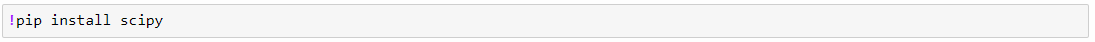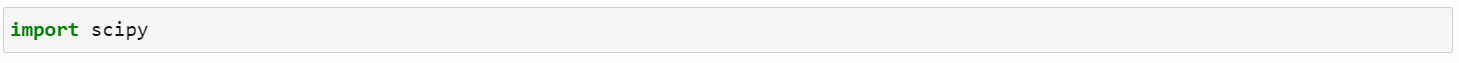• Via Anaconda Prompt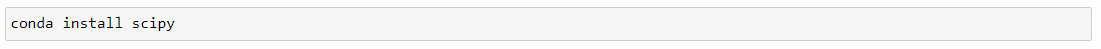## How to upgrade to the new version?

SciPy module in Python gets upgraded with new releases. As of Jun 28, 2023, the release is the 1.11.1 version.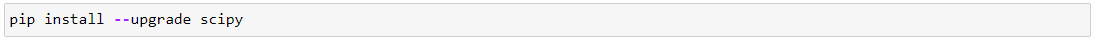## Understand more about SciPy through Python:

Python has inbuilt functions that retrieve information/guidelines about the packages & functions and the parameters concerning them.

Below are two must-know functions used to quickly refer to any package.

• dir will provide us with a list of methods available on the object of interest
• help will inspect a specific method we would like to use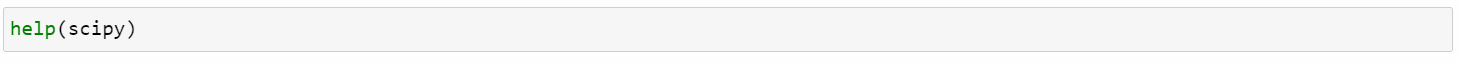## Sub-Packages:

SciPy has multiple sub-packages with various functionalities. It can be imported as shown below: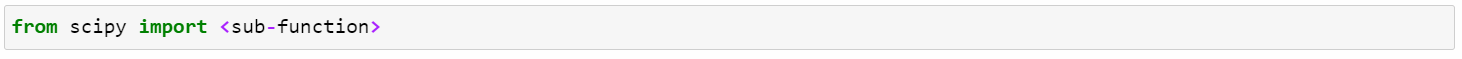Example: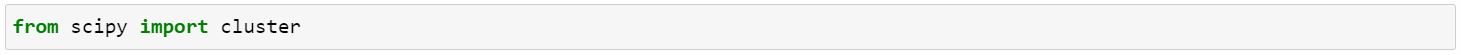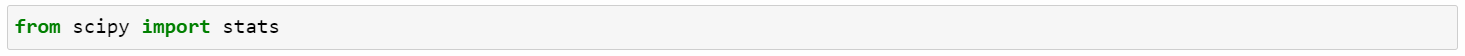Import Packages: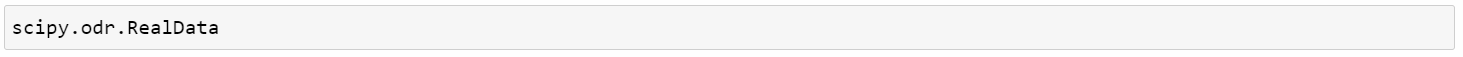Or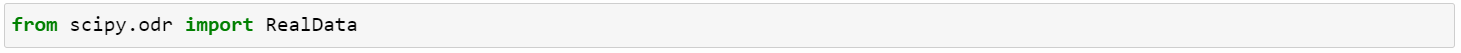## SciPy Python Library: Packages and its uses:

No. Packages Description
1 scipy.cluster Various clustering algorithms like Kmeans, Hierarchical, and DBSCAN
2 scipy.constants Collection of physical and mathematical constants used in scientific calculations
3 scipy.datasets Collection of pre-loaded datasets that are commonly used in experimenting with various scientific tasks
4 scipy.fft Fast Fourier Transform
5 scipy.integrate Perform integration
6 scipy.interpolate Provides functions for interpolating data (estimating values between own data points)
7 scipy.io Reading and writing data in various formats (MATLAB,WAV,NetCDF, image files, etc
8 scipy.linalg Linear algebra operations
10 scipy.ndimage Image processing and data manipulation
11 scipy.odr Interface to Orthogonal Distance Regression (ODR) algorithm
12 scipy.optimize Optimization and root finding algorithms
13 scipy.signal Signal Processing
14 scipy.sparse Works with sparse matrices
15 scipy.spatial Spatial data structures and algorithms
16 scipy.special Evaluation of mathematical special functions
17 scipy.stats Statistical functions and normal distribution

Looking forward to becoming a Python Trainer? Check out the Python Course in Bangalore and get certified today.

## Commonly used packages: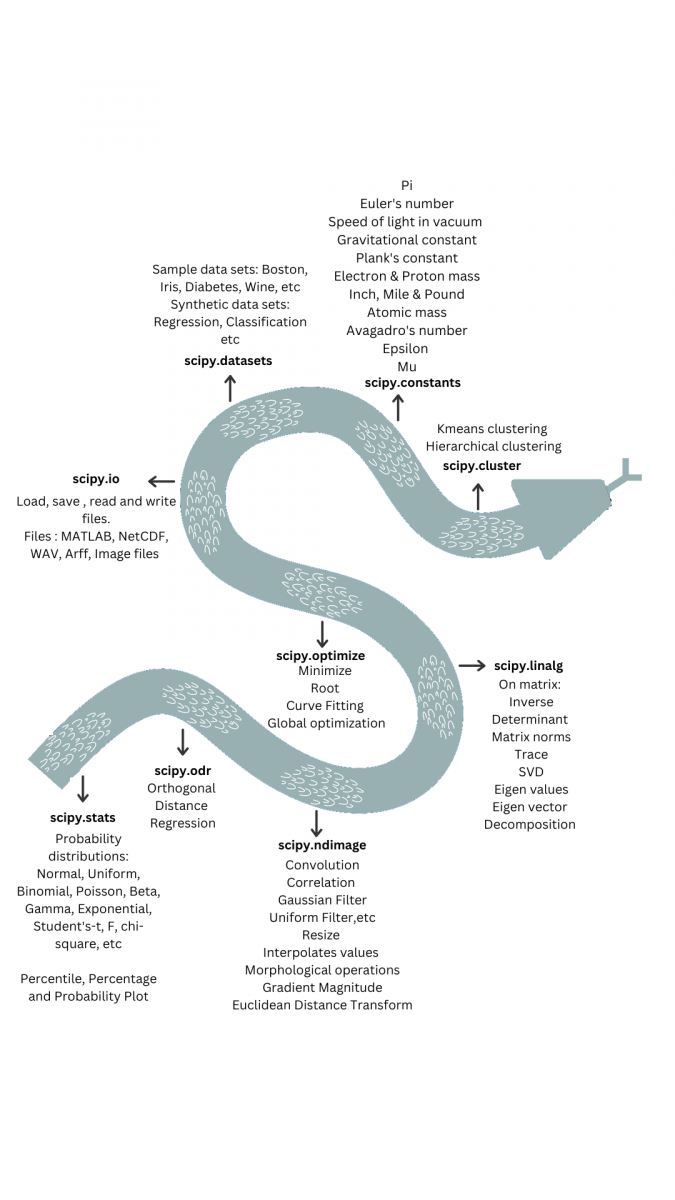## A quick reference to various functions in SciPy:

The SciPy program in Python has many functions and sub-functions. Here, listed are a few commonly used functions:

• Boxcox
• IQR
• Constants
• Datasets
• Access Files
• Normal Distribution

#### First Business Moment: Mean, Median and Mode

The formula for mean, median and mode is: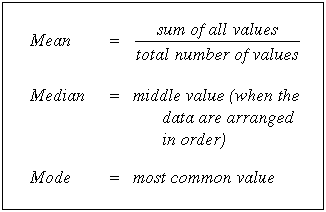Import packages:Or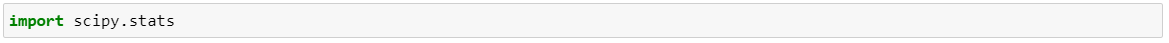Mean:

mean()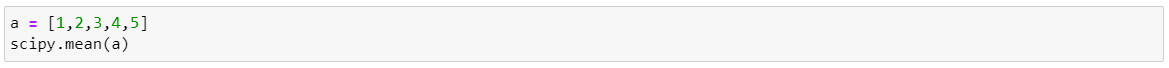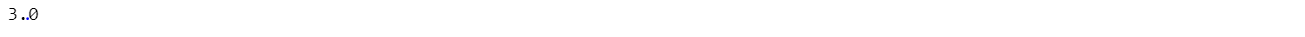Median:

median()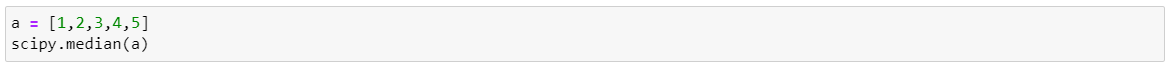Mode:

mode()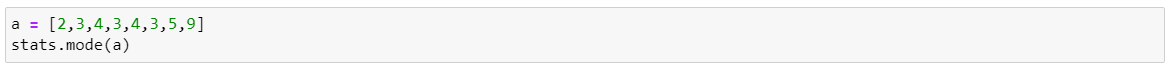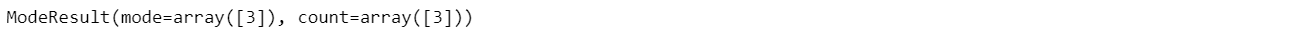#### Second Business Moment: Variance, Standard Deviation

The formula for variance and standard deviation is: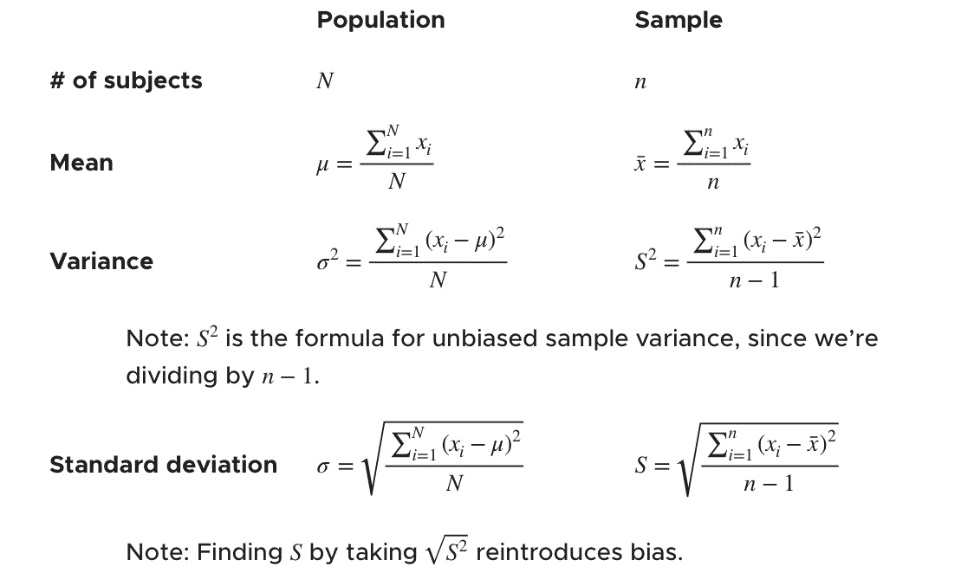Variance:

var()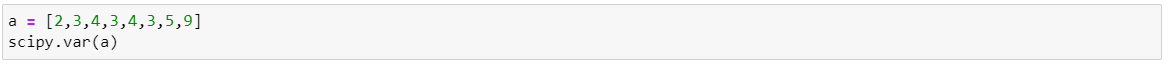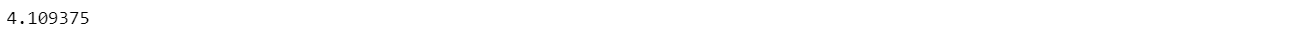Standard deviation:

Std()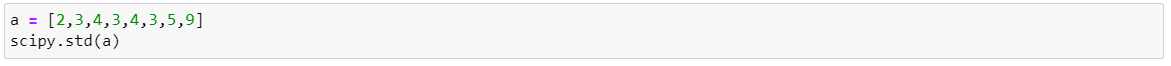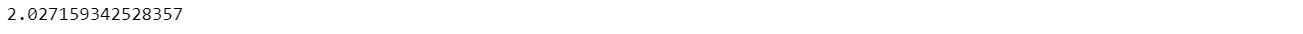The formula for skewness is: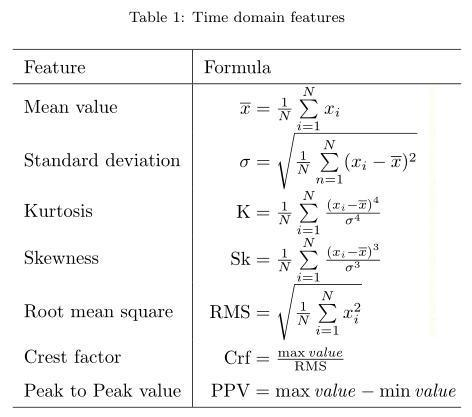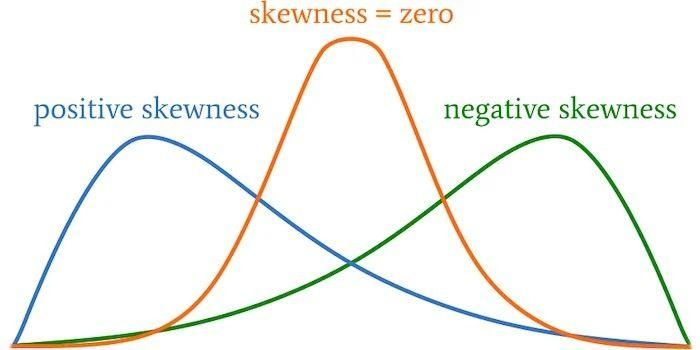skew()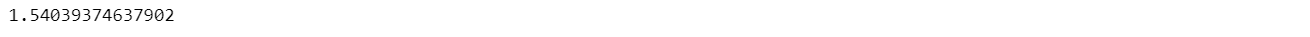The formula for Kurtosis is: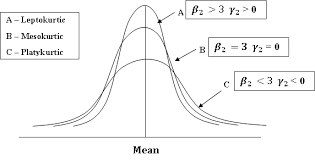kurt()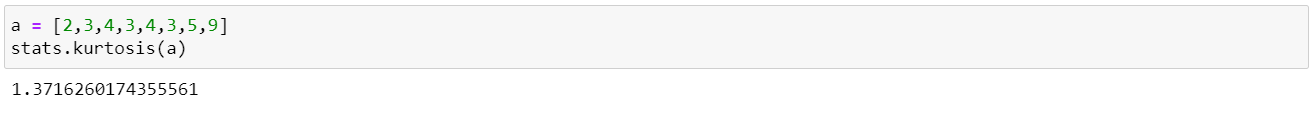## 2. Boxcox:

SciPy package in Python has Boxcox function which is used to transform non-normal data into normal data.

Required packages are imported.

A random non-normal data (exponential distribution) set is created.

Boxcox() function is used to transform the data to normal distribution.

The lambda value used for transformation is found.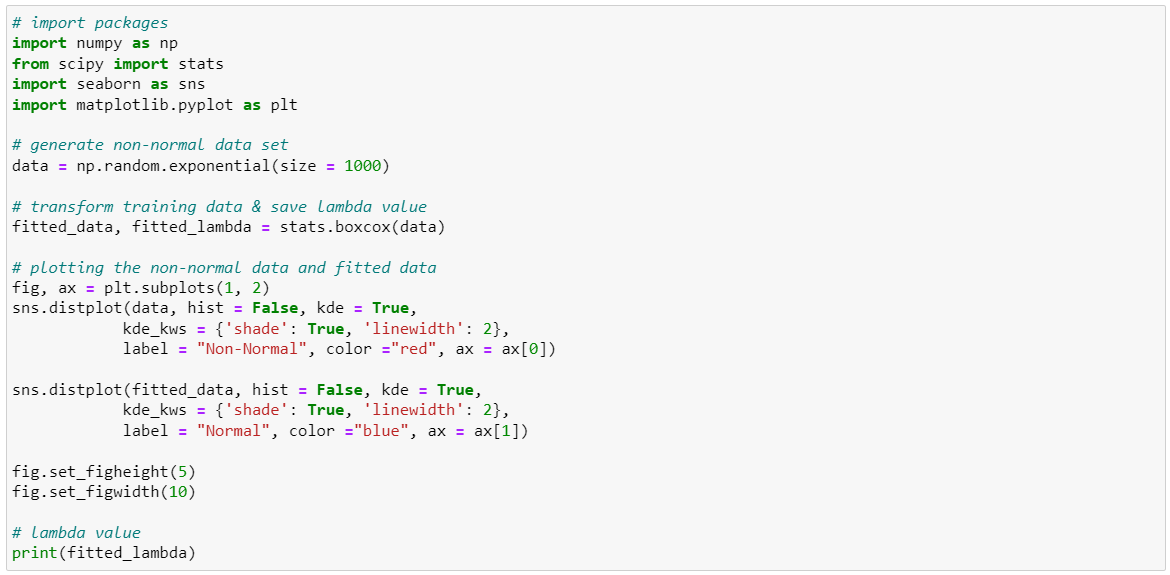The non-normal and normal data are shown below: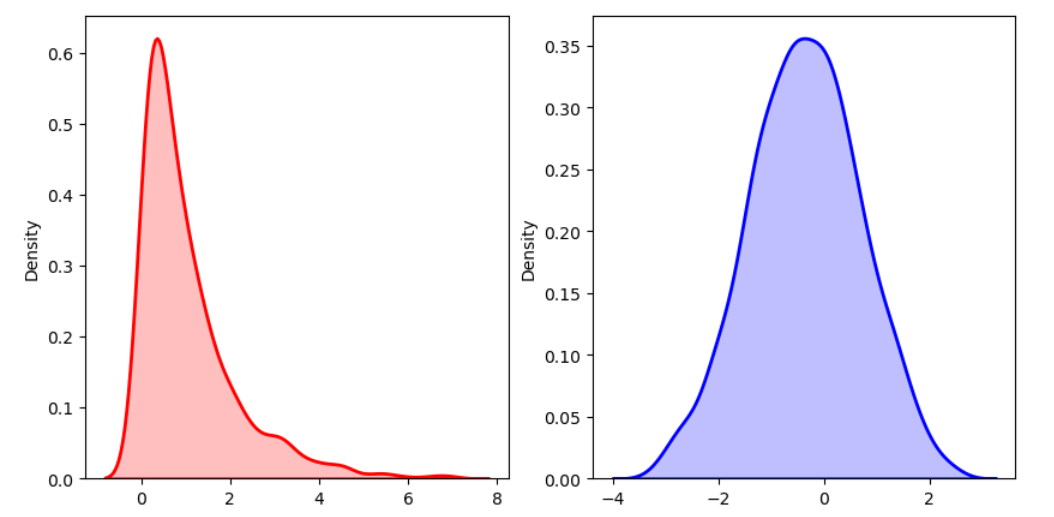The Lambda value used to normalize the data is 0.238934.

## 3. IQR: Interquartile Range

The formula for the Interquartile range is:

Interquartile range = Upper Quartile – Lower Quartile = Q3 – Q1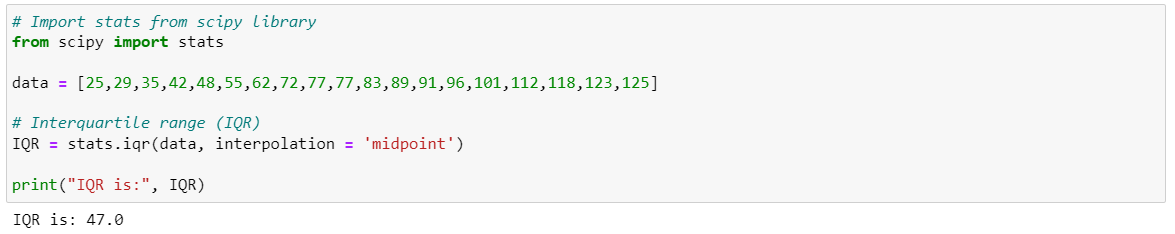## 4. Constants

SciPy provides various physics and mathematical constants which are used in scientific calculations.

Below is the list of constants: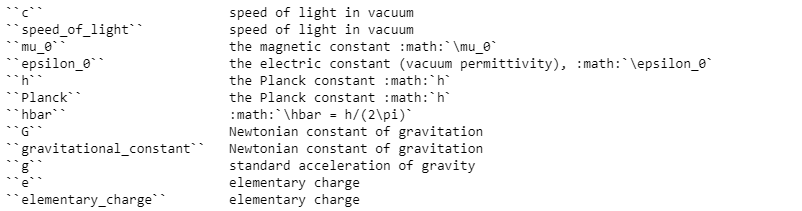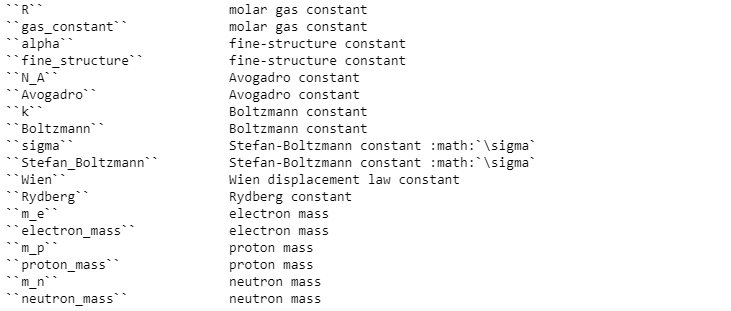#### Constant Values

The code to find the constant value is shown in the below example.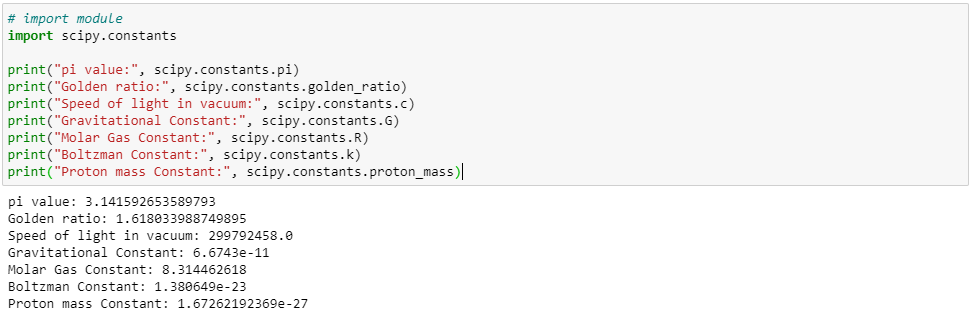Search / Find

The below finds out all the constants containing the 'proton' word in it and returns a list of constants.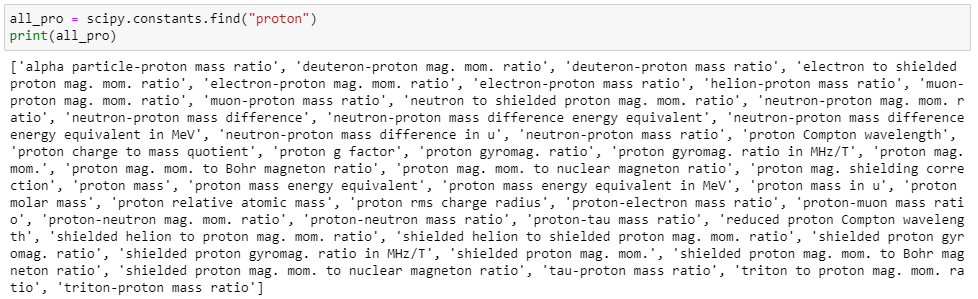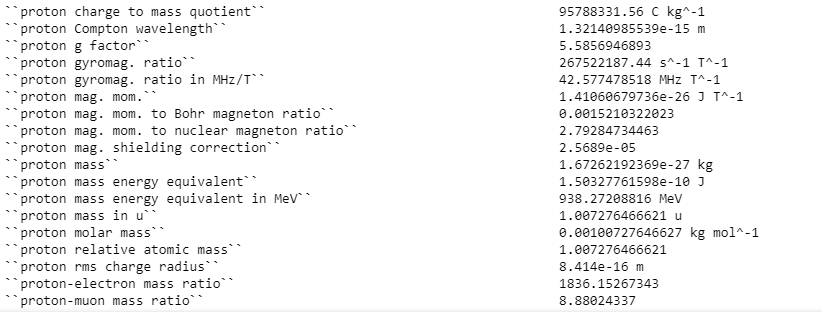Value with Unit and Degree of Uncertainty

Along with the magnitude of the constant, we can also access the unit and degree of uncertainty###### Example of how to use constant:

Area of circle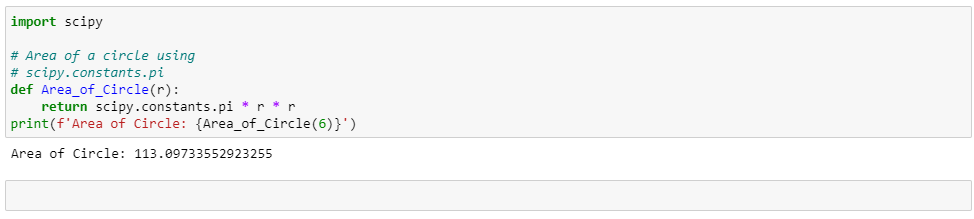## 5. Datasets:

Get a color image of a raccoon face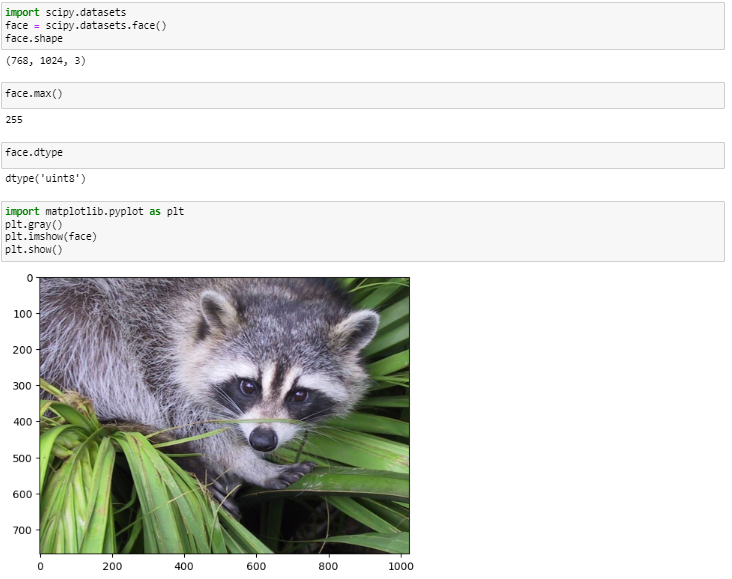## 6. Access File

Here are some ways to load and read a file using scipy.io.

The scipy.io module is part of the SciPy library and provides functions for working with various file formats used in scientific computing, such as MATLAB files, NetCDF files, and WAV files.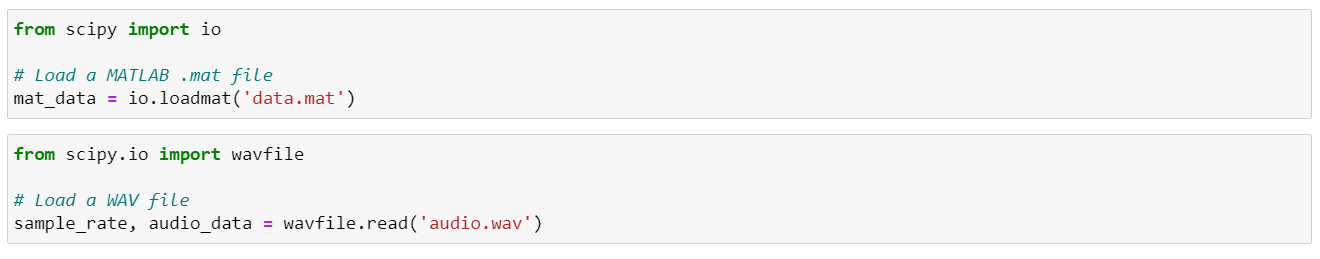## 7. Normal Distribution

Python SciPy library helps generate all kinds of normal distribution. The normal distribution is the symmetrical distribution of data around its mean value. It is also called the Bell Curve.

The object norm() in scipy.stats function is used to generate normal distributions. The parameters are data, size, moments loc and scale.

The normal distribution depends on the mean and standard deviation. Hence the loc is for the mean and the scale is for the standard deviation.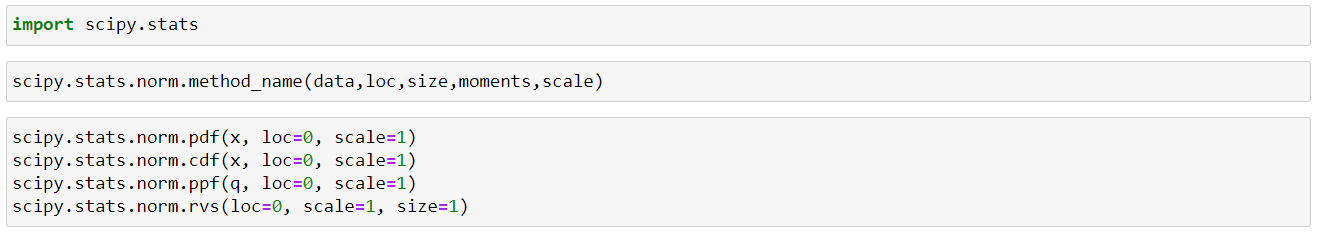##### Probability Distribution Function:

Method 1:

The mean and standard deviation values are entered manually.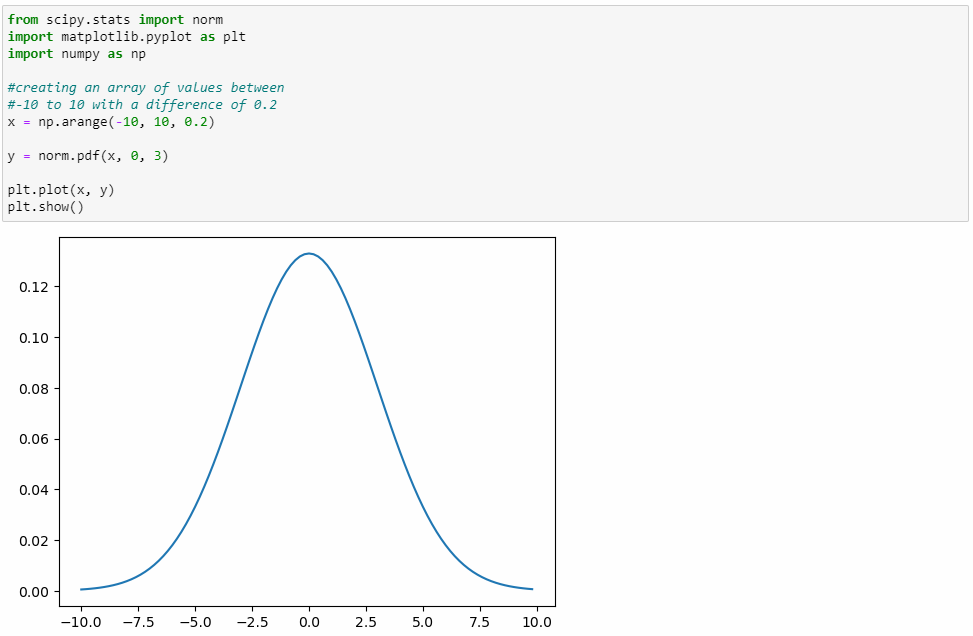Method 2:

The mean and standard deviation values are calculated using Numpy and then used to plot.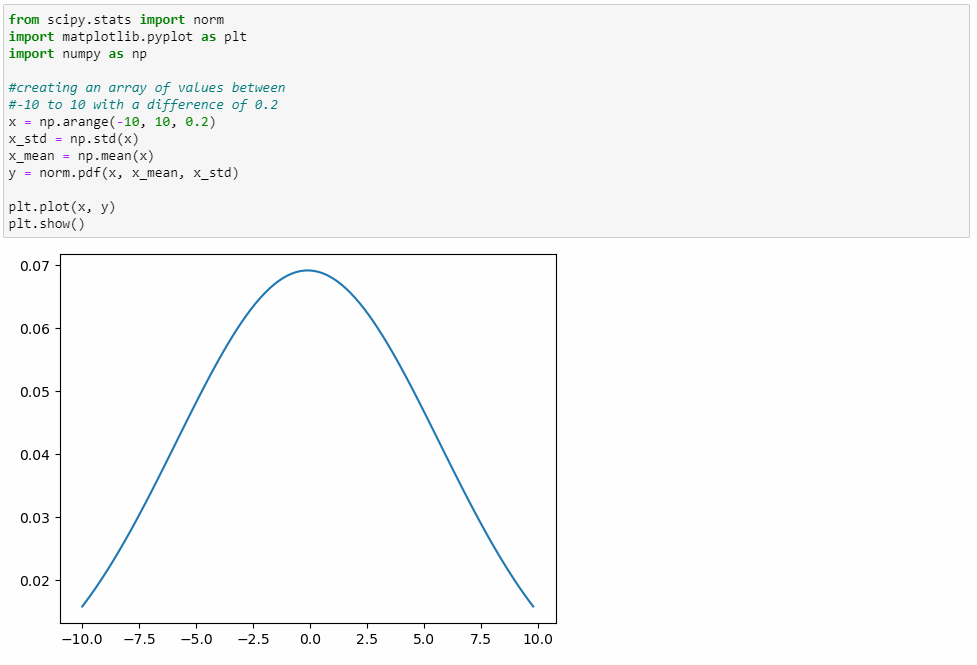##### Cumulative Distribution Function:

Method 1:

The mean and standard deviation values are entered manually.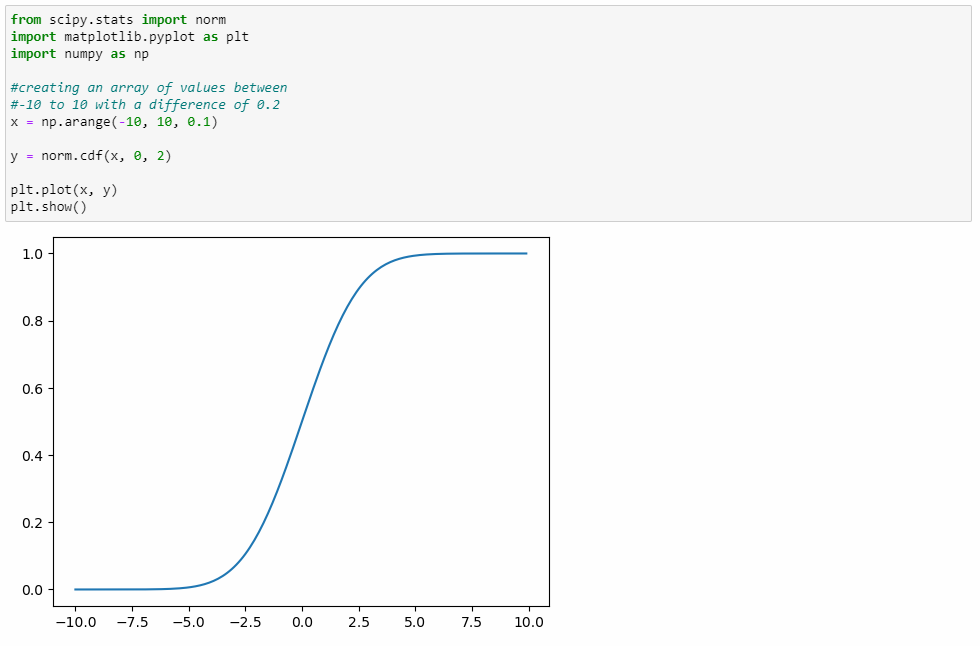Method 2:

The mean and standard deviation values are calculated using Numpy and then used to plot.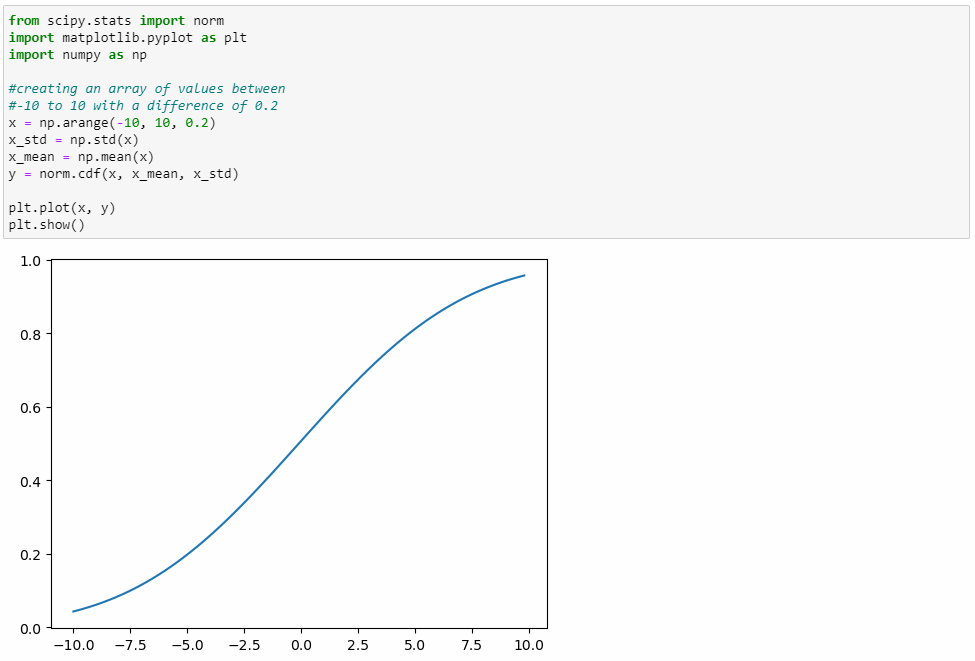Random Variates:

Random Variate in norm object returns the specified number of random numbers in the normal distribution.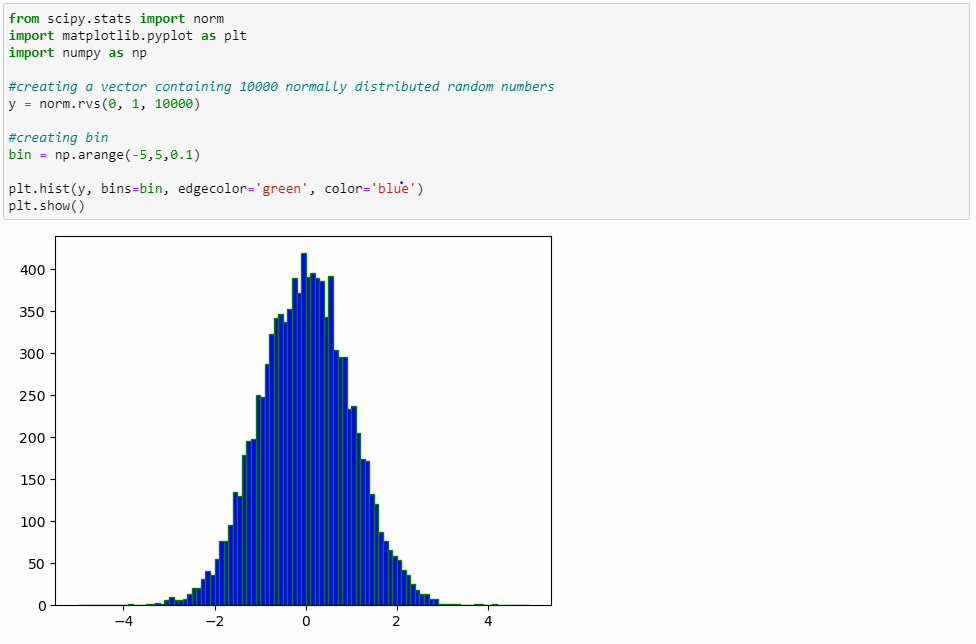Probability Point Function: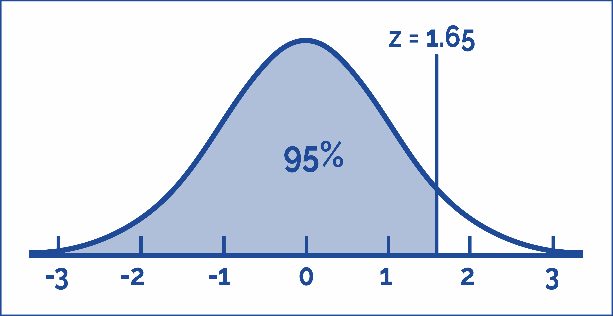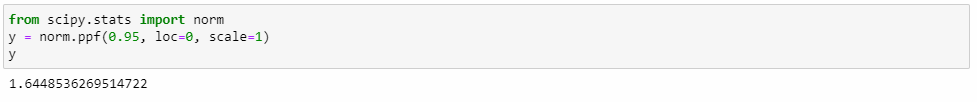Before we conclude, let's have a little interactive.

360DigiTMG offers the Best Python Training in Chennai to start a career in Python Training. Enroll now!

Imagine you are a scientist working on a fascinating project, and you need to utilize SciPy to solve a critical problem. In this scenario, you're investigating the spread of a deadly virus in a densely populated city. You have data on the virus's transmission rates, population density, and other factors. Your task is to use SciPy to create a predictive model and find the best strategy to control the virus's spread.In the comments section below, let's brainstorm and interactively build the steps for this critical task using SciPy. Feel free to contribute ideas, ask questions, or suggest different approaches.

### Data Analyst Courses in Other Locations